Function Repository Resource:

# PointLineDistance

Get the distance and normal line between a point and line

Contributed by: Ed Pegg Jr
 ResourceFunction["PointLineDistance"][p,{a,b}] returns the distance between the point p and the infinite line through points a and b, as well as the coordinates realizing that distance. ResourceFunction["PointLineDistance"][Point[p],Line[{a,b}]] returns the distance between point p and the infinite line through points a and b, as well as the Line realizing that distance.

## Examples

### Basic Examples (2)

Calculate distance and normal line for (1,1) and the x-axis:

 In:=Out=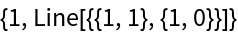Show the construction:

 In:=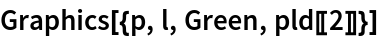Out=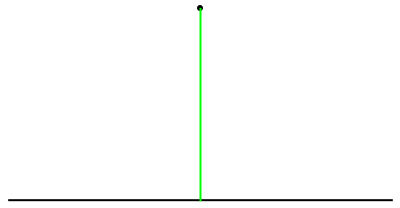For just the distance, RegionDistance can be used:

 In:=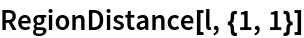Out=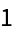For the point on the line, RegionNearest can be used:

 In:=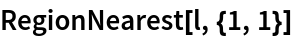Out=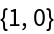For a more arbitrary point, the line is assumed to be infinite:

 In:=Out=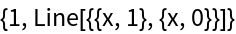With RegionNearest, InfiniteLine must be specified:

 In:=Out=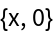### Scope (2)

Using Point and Line is optional:

 In:=Out=Use PointLineDistance on a point and line in 3D:

 In:=Out=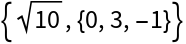### Applications (2)

Plot a tilted cylinder:

 In:=Out=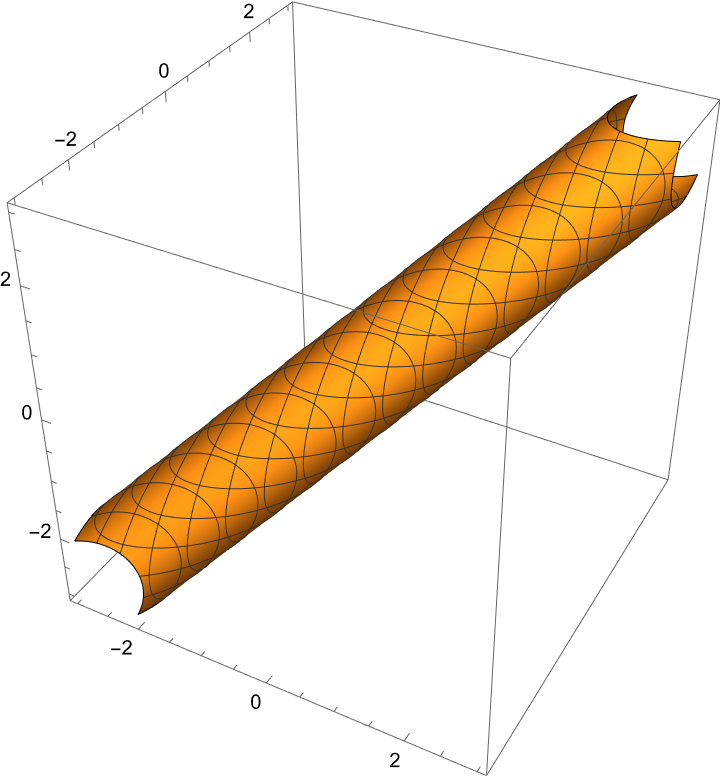A parabola can be defined as the locus of points that are equidistant between a given point (the focus) and a given line (the directrix). Plot a parabola, given a focus and directrix:

 In:=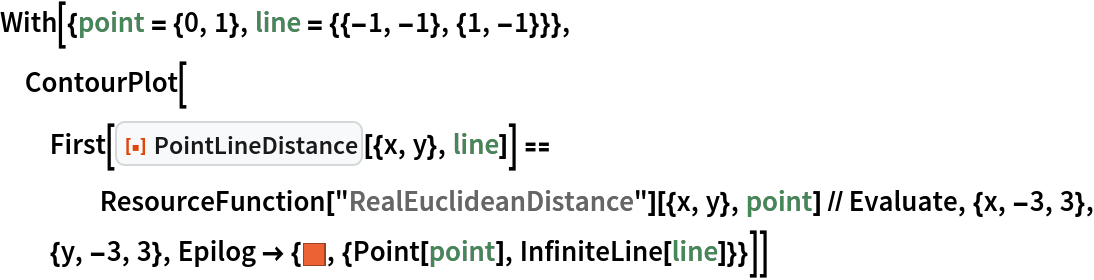Out=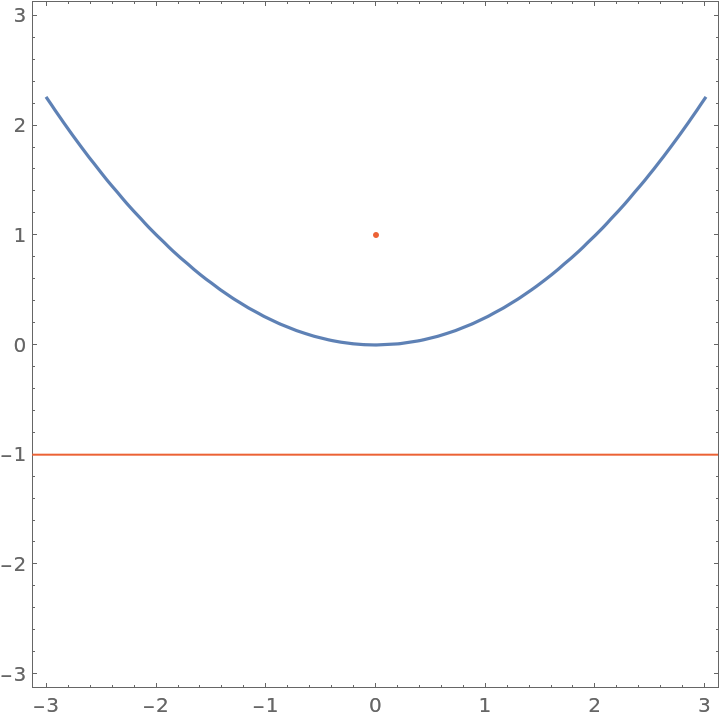## Version History

• 1.0.0 – 18 April 2022